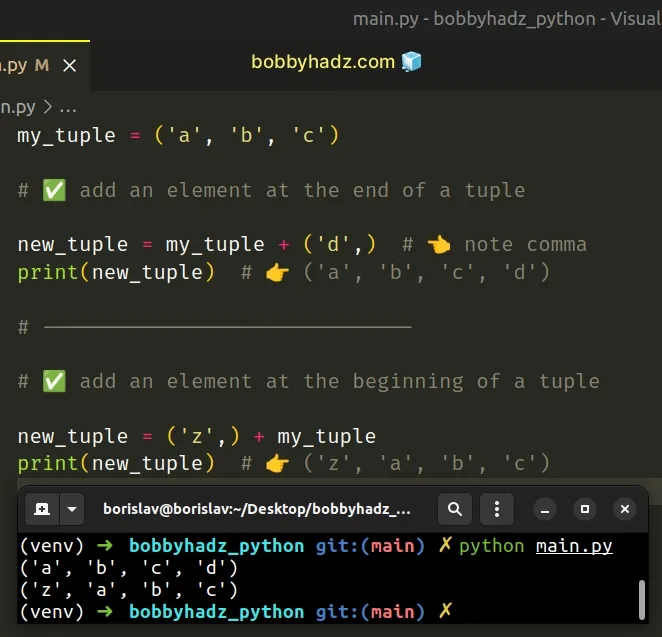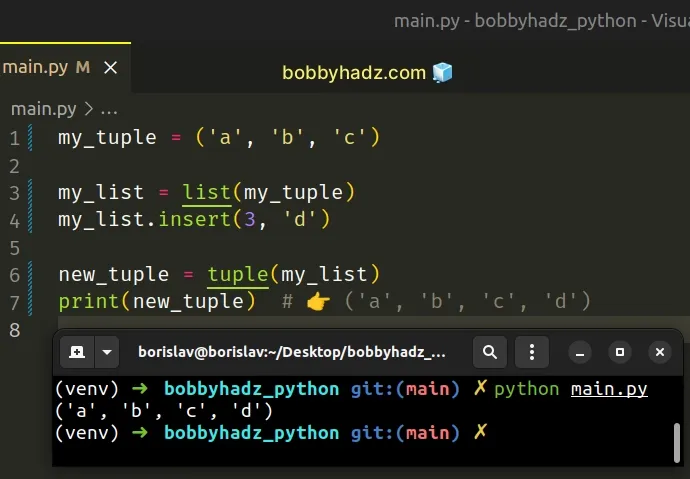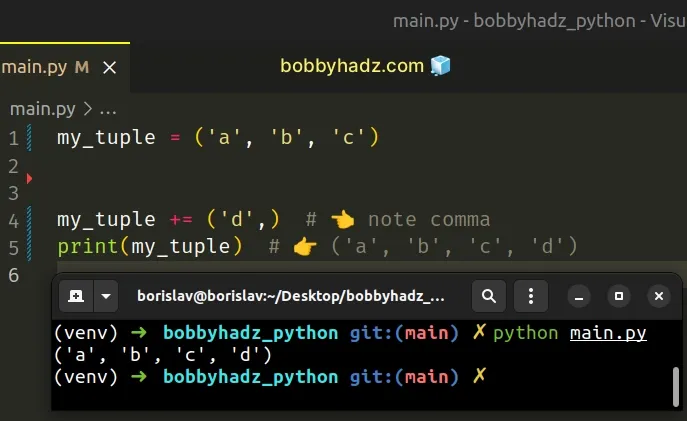# Insert/Append an Element into a Tuple in PythonLast updated: Feb 19, 2023
5 min## #Append an element to a Tuple using the addition (+) operator

Use the addition (+) operator to append an element to a tuple, e.g. `new_tuple = my_tuple + ('new', )`.

Tuples are immutable, so to append an element into a tuple, we have to create a new tuple that contains the element.

main.py
```Copied!```my_tuple = ('a', 'b', 'c')

# ✅ add an element at the end of a tuple

new_tuple = my_tuple + ('d',)  # 👈️ note comma
print(new_tuple)  # 👉️ ('a', 'b', 'c', 'd')

# ------------------------------

# ✅ add an element at the beginning of a tuple

new_tuple = ('z',) + my_tuple
print(new_tuple)  # 👉️ ('z', 'a', 'b', 'c')
``````The same approach can be used to append multiple elements to a tuple.

main.py
```Copied!```my_tuple = ('a', 'b', 'c')

new_tuple = my_tuple + ('d', 'e')
print(new_tuple)  # 👉️ ('a', 'b', 'c', 'd', 'e')
``````

Notice that we wrapped the value in parentheses and added a trailing comma, so that the values on the left-hand and right-hand sides of the addition (+) operator are tuples.

The trailing comma is significant and is the difference between creating a tuple and a string.
main.py
```Copied!```print(type(('a',)))  # 👉️ <class 'tuple'>

print(type(('a')))  # 👉️ <class 'str'>
``````

Tuples are constructed in multiple ways:

• Using a pair of parentheses `()` creates an empty tuple
• Using a trailing comma - `a,` or `(a,)`
• Separating items with commas - `a, b` or `(a, b)`
• Using the `tuple()` constructor

## #Insert an element into a Tuple in Python

If you need to insert an element into a tuple:

1. Use the `list()` class to convert the tuple to a list.
2. Use the `list.insert()` method to insert the element into the list.
3. Use the `tuple()` class to convert the list back to a tuple.
main.py
```Copied!```my_tuple = ('a', 'b', 'c')

my_list = list(my_tuple)
my_list.insert(3, 'd')

new_tuple = tuple(my_list)
print(new_tuple)  # 👉️ ('a', 'b', 'c', 'd')
``````Tuples are very similar to lists, but implement fewer built-in methods and are immutable (cannot be changed).

Since tuples cannot be changed, the only way to insert an element into a tuple is to create a new tuple that contains the element.

The example converts the tuple to a list, uses the `list.insert()` method and converts the list back to a tuple.

main.py
```Copied!```my_tuple = ('a', 'b', 'c')

my_list = list(my_tuple)
my_list.insert(3, 'd')

new_tuple = tuple(my_list)
print(new_tuple)  # 👉️ ('a', 'b', 'c', 'd')
``````

The list class takes an iterable and returns a list object.

The `list.insert` method inserts an item at a given position.

The method takes the following 2 parameters:

NameDescription
indexThe index of the element before which to insert
itemThe item to be inserted at the given index

In the example, we added the string `d` at index `3` and used the tuple() class to convert the list back to a tuple.

## #Append an element to a Tuple using reassignment

Alternatively, you can use reassignment.

main.py
```Copied!```my_tuple = ('a', 'b', 'c')

my_tuple += ('d',)  # 👈️ note comma
print(my_tuple)  # 👉️ ('a', 'b', 'c', 'd')
``````This approach is useful when you don't need to keep access to the value of the tuple prior to appending the element.

Instead of declaring a variable that stores the new tuple, we assign a new value to the original variable.

## #Append an element to a Tuple using iterable unpacking

You can also unpack the tuple into a new tuple.

main.py
```Copied!```my_tuple = ('a', 'b', 'c')

new_tuple = (*my_tuple, 'd')

print(new_tuple) # 👉️ ('a', 'b', 'c', 'd')
``````

The * iterable unpacking operator enables us to unpack an iterable in function calls, in comprehensions and in generator expressions.

main.py
```Copied!```example = (*(1, 2), 3)

# 👇️ (1, 2, 3)
print(example)
``````

The values from the tuple get unpacked into a new tuple where we can add extra elements.

## #Insert an element into a tuple using slicing

Alternatively, you can use tuple slicing.

main.py
```Copied!```def insert_into_tuple(tup, index, value):
new_tuple = tup[:index] + (value,) + tup[index:]

return new_tuple

my_tuple = ('a', 'b', 'd')

# 👇️ ('a', 'b', 'c', 'd')
print(insert_into_tuple(my_tuple, 2, 'c'))

# 👇️ ('z', 'a', 'b', 'd')
print(insert_into_tuple(my_tuple, 0, 'z'))
``````

The `insert_into_tuple` function takes a tuple, an index and a value as parameters and inserts the value into the tuple at the specified index.

The syntax for tuple slicing is `my_tuple[start:stop:step]`.

The `start` index is inclusive and the `stop` index is exclusive (up to, but not including).

If the `start` index is omitted, it is considered to be `0`, if the `stop` index is omitted, the slice goes to the end of the tuple.

Python indexes are zero-based, so the first item in a tuple has an index of `0`, and the last item has an index of `-1` or `len(my_tuple) - 1`.

The slice `tuple[:index]` starts at index `0` and goes up to, but not including the specified index.

main.py
```Copied!```def insert_into_tuple(tup, index, value):
new_tuple = tup[:index] + (value,) + tup[index:]

return new_tuple
``````

The slice `tuple[index:]` starts at the specified index and goes to the end of the tuple.

Notice that we added a trailing comma after the value we want to insert into the tuple.

It is necessary to wrap the value in a tuple to be able to use the addition (+) operator.

## #Append an element to a tuple using conversion to list

You can also convert the tuple to a list and use the `append` method.

main.py
```Copied!```
my_tuple = ('a', 'b', 'c')

my_list = list(my_tuple)

my_list.append('d')
print(my_list) # 👉️ ['a', 'b', 'c', 'd']

my_tuple = tuple(my_list)
print(my_tuple) # 👉️ ('a', 'b', 'c', 'd')
``````

The code sample uses the `list()` class to convert the tuple to a list and appends elements to the list.

You can optionally convert the list back to a tuple by using the `tuple()` class.

If you need to append multiple values to the tuple, use the `list.extend()` method.

main.py
```Copied!```my_tuple = ('a', 'b', 'c')

my_list = list(my_tuple)

my_list.extend(['d', 'e'])
print(my_list)  # 👉️ ['a', 'b', 'c', 'd', 'e']

my_tuple = tuple(my_list)
print(my_tuple)  # 👉️ ('a', 'b', 'c', 'd', 'e')
``````